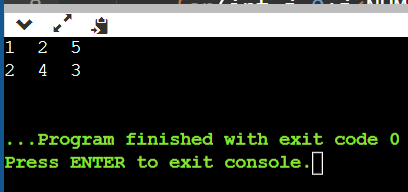# Find the transpose of a 2D matrix

## Question

Write a function that takes two 2D arrays, data and dataT, as parameters. Assume that NUMROWS and NUMCOLS have been defined to be the number of rows and number of columns in the array data, respectively. Further, the number of rows in data is equal to the number of columns in dataT and the number of columns in data is equal to the number of rows in dataT. At the end of the function call, column “i” of dataT should be equal to row “i” of data, for each “i” from 0 to (NUMROWS 1).

If you’ve taken a linear algebra course, think of data and dataT as two-dimensional matrices, in which case dataT will be the transpose of data when this function has been executed.

For example, if data corresponds to the array

{{1, 2}, { 2, 4 }, {5, 3} },

then after the function call, dataT must correspond to the array

{{1, 2, 5 }, { 2, 4, 3} }.

Here is the function prototype:

void transpose( int data [] [NUMCOLS], int dataT[] [NUMROWS] );

## Summary

In this question, we have to write a C++ program in which we will take one 2D array (data) and we will print the transpose of that 2D array (dataT). We have given the number of rows and columns present in the data matrix. Here we have taken a matrix of size 3X2, and the transpose of the matrix will be of size 2X3.
data = 1 2
2 4
5 3

dataT = 1 2 5
2 4 3
We have defined a function with the name transpose in which we have passed both matrixes, and then we have updated the matrix dataT. We will be transposing a matrix.

## Explanation

Here we have declared two global variables with the name NUMROWS and NUMCOLS and we have assigned the values 3 and 2 respectively.

In the main method, we have declared the 2D array with the name data of size 3X2. Also, we have declared a 2D array of size 2X3 with the name dataT in which, we will store the transpose of the data matrix.

We have passed both 2D arrays to the function transpose.

We have defined a function with the name transpose. In the transpose function, we have traversed every element and kept it in its desired location in dataT matrix. At last, we will display the 2D array dataT to the console as output, element by element.

## Code

#include <iostream>
using namespace std;
/* to store the number of rows and columns */
int NUMROWS=3, NUMCOLS=2;
/* function to transpose a matrix */
void transpose(int data, int dataT){
for(int i=0;i<NUMROWS;i++){
for(int j=0;j<NUMCOLS;j++){
dataT[j][i]=data[i][j];
}
}
/* to print the resultant transposed matrix */
for(int i=0;i<NUMCOLS;i++){
for(int j=0;j<NUMROWS;j++){
cout<<dataT[i][j]<<"  ";
}
cout<<endl;
}
}
/* driver code */
int main()
{
int data={{1,2},{2,4},{5,3}};
int dataT;
transpose(data,dataT);
}


## Output

After transposing a matrix, the output is: